Next: Maximum-entropy priors Up: General principle based priors Previous: General principle based priors

### Transformation invariance

An important class of priors arises from the requirement of transformation invariance. We shall consider two specific cases, translation invariance and scale invariance.
Translation invariance

Let us assume we are indifferent over a transformation of the kind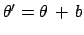, where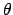is our variable of interest and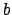a constant. Thenis an infinitesimal mass element of probability forto be in the interval. Translation invariance requires that this mass element remains unchanged when expressed in terms of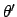, i.e.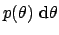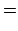(100)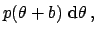(101)

since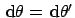. It is easy to see that in order for Eq. (101) to hold for any,must be equal to a constant for all values offrom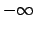to. It is therefore an improper prior. As discussed above, this is just a convenient modelling. For practical purposes this prior should always be regarded as the limit forof, where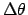is a large finite range around the values of interest.

Scale invariance

In other cases, we could be indifferent about a scale transformation, that is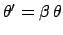, where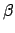is a constant. This invariance implies, since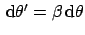in this case,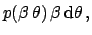(102)

i.e.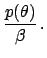(103)

The solution of this functional equation is(104)

as can be easily proved using Eq. (104) as test solution in Eq. (103). This is the famous Jeffreys' prior, since it was first proposed by Jeffreys. Note that this prior also can be stated as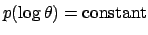, as can be easily verified. The requirement of scale invariance also produces an improper prior, in the range. Again, the improper prior must be understood as the limit of a proper prior extending several orders of magnitude around the values of interest. [Note that we constrainto be positive because, traditionally, variables which are believed to satisfy this invariance are associated with positively defined quantities. Indeed, Eq. (104) has a symmetric solution for negative quantities.]
According to the supporters of these invariance motivated priors (see e.g. Jaynes 1968, 1973, 1998, Sivia 1997, and Fröhner 2000, Dose 2002) variables associated to translation invariance are location parameters, as the parameterin a Gaussian model; variables associated to scale invariance are scale parameters, like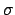in a Gaussian model or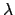in a Poisson model. For criticism about the (mis-)use of this kind of prior see (D'Agostini 1999d).Next: Maximum-entropy priors Up: General principle based priors Previous: General principle based priors
Giulio D'Agostini 2003-05-13